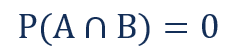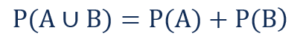# Mutually Exclusive Events

Two events that cannot occur at the same time

## What are Mutually Exclusive Events?

In statistics and probability theory, two events are mutually exclusive if they cannot occur at the same time. The simplest example of mutually exclusive events is a coin toss. A tossed coin outcome can be either head or tails, but both outcomes cannot occur simultaneously.Mutually exclusive events are commonly confused with independent events. However, they are two distinct concepts. Unlike mutually exclusive events, independent events can occur simultaneously. The independence of the events indicates that the outcome probability of one event does not influence the outcome probability of another event.

### Mutually Exclusive Events in Finance

The concept of mutually exclusive events offers numerous applications in finance. Such events are frequently encountered during the decision-making process in corporate finance. For example, capital budgeting processes consider mutually exclusive long-term investment projects.

In addition, mutually exclusive events can be found in investment management. For instance, due to certain constraints, the portfolio manager could face limited investment opportunities. If some of the opportunities cannot be employed together, they are recognized as mutually exclusive.

In finance, the analysis of the events considers both statistical and financial aspects. Besides the probabilities of the events, the analysis generally includes various financial metrics such as the Net Present Value (NPV), returns or some macroeconomic factors.

### Probability Rules for Mutually Exclusive Events

Despite the specific features of mutually exclusive events, the events still follow some of the fundamental probability rules. The rules include the following:

#### 1. Rule of Multiplication

The rule of multiplication is used when we want to find the probability of events occurring simultaneously (it is also known as the joint probability of independent events). The rule of multiplication for mutually exclusive events states the following:Since the events cannot occur simultaneously, their joint probability is always zero.

The rule of addition allows determining the probability that at least one of the events occurs (it is known as the union of the events). For mutually exclusive events, the rule of addition defines the following:The union probability of the events is found by summing only the individual probabilities of each event because there is a zero probability that both events can occur at the same time.